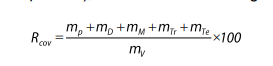# Lesson 5: RECYCLABILITY AND RECOVERABILITY RATES – CALCULATION OF THE RECYCLABILITY AND RECOVERABILITY RATE

In this lesson, we will dive into the calculation of the recyclability and recoverability rates for end-of-life vehicles.

1. Recyclability Rate
• The Recyclability rate (Rcyc) of a vehicle is expressed as a percentage by mass, denoted as a mass fraction in percent.
• The calculation for Rcyc is as follows:
• Where:
• Mp is the mass determined in the preliminary preparation phase.
• Md is the mass determined during dismantling.
• Mm is the mass of metals determined during metal separation.
• Mtp is the mass of non-metallic residues considered recyclable.
• Rcyc is presented as a percentage (%).
1. Recoverability Rate
• The Recoverability rate (Rcov) of a vehicle is also expressed as a percentage by mass, denoted as a mass fraction in percent.
• The calculation for Rcov is as follows:Where:

• Mp is the mass determined in the preliminary preparation phase.
• Md is the mass determined during dismantling.
• Mm is the mass of metals determined during metal separation.
• Mtp is the mass of non-metallic residues considered recyclable.
• Mte is the mass of remaining materials that could potentially be used for energy renewal.
• Rcov is presented as a percentage (%).
1. Rounding Values
• Given the high required threshold values (Rcyc ≥ 85%, Rcov ≥ 95%), manufacturers aim to achieve values close to these thresholds.
• To display these values, one decimal place is typically used with the following rounding rules:
• If the number behind the decimal point is between 0 and 4, it is rounded down to the nearest integer value.
• If the number behind the decimal point is between 5 and 9, it is rounded up to the nearest integer value.
1. Formalized Data Overview
• Table 3 provides a formalized overview of data obtained through the calculation method. This overview is defined in accordance with the ISO 22628:2002 standard and is an essential part of the manufacturer’s documentation submitted to the Competent State Authority for homologation.
1. Presentation of Data
• Figure 11 illustrates the presentation of data for a specific vehicle. This type of data presentation is also an integral part of the Approval Certificate, as required by Directive 2005/64/EC or UN Regulation No. 133.

Conclusion

• Calculating the recyclability and recoverability rates is essential for assessing the environmental impact and sustainability of end-of-life vehicles.
• Manufacturers strive to achieve high rates to meet stringent regulations, and the values are typically expressed with one decimal place, following specific rounding rules.